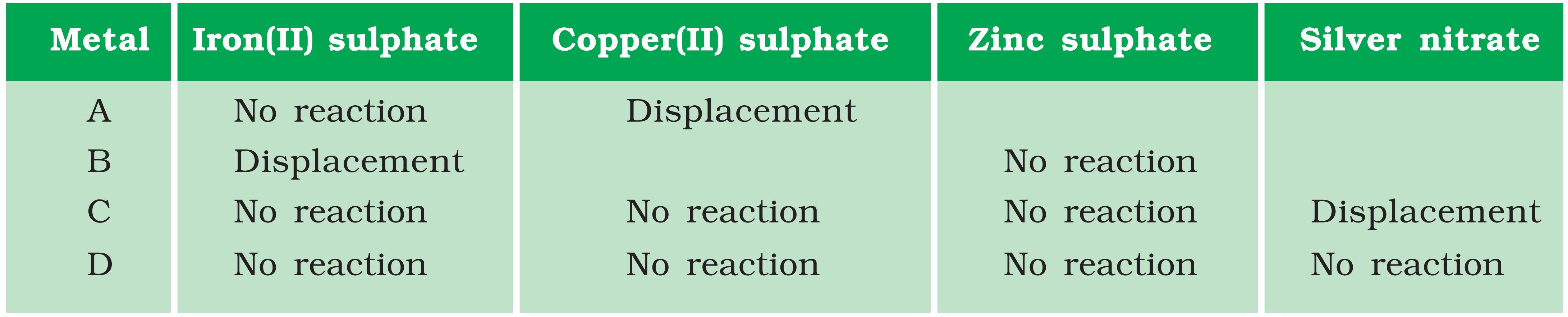Questions from inside the book

Class 10
Chapter 3 Class 10 - Metals and Non-Metals (Term 1)

# Samples of four metals A, B, C and D were taken and added to the following solution one by one. The results obtained have been tabulated as follows.## (iii) Arrange the metals A, B, C and D in the order of decreasing reactivity.

(i)

According to the reactivity series , among the 4 salts given, Zinc Sulphate is the most reactive

• Since no metals react with it, we consider the next most reactive solution, Iron (II) Sulphate .
• Since only B is able to displace Iron from Iron (II) Sulphate, it is the most reactive among A,B,C,D

(ii) What would you observe if B is added to a solution of Copper(II) sulphate?

B is more reactive than Fe, which in turn is more reactive than Cu.

And so, B will displace Cu from its salt solution.

(iii) Arrange the metals A, B, C and D in the order of decreasing reactivity.

Let us go in order of most reactive to least reactive salt solutions.

• Zinc solution is the most reactive salt solution here. But no metal reacts with it
• B is the most reactive element as it is able to displace Iron from its salt solution .
• A is able to displace copper from its salt solution.
• C is able to displace Silver from its salt solution.
• D is the least reactive element, since it does not react at all .

So,  The reactivity series in decreasing order is given as

B>A>C>D

983 students joined Teachoo Black. What are you waiting for?